[BACK]
 Computer Systems Science & EngineeringDOI:10.32604/csse.2022.019298Article

Network Traffic Prediction Using Radial Kernelized-Tversky Indexes-Based Multilayer Classifier

1Department of Computer Applications, Velalar College of Engineering and Technology, Erode, 638012, India
2Department of Electronics and Communication Engineering, Velalar College of Engineering and Technology, Erode, 638012, India
3Department of Information Technology, Kongu Engineering College, Perundurai, India
*Corresponding Author: M. Govindarajan. Email: mgovindarajan2021@gmail.com, govind.mothilal@gmail.com
Received: 09 April 2021; Accepted: 15 May 2021

Abstract: Accurate cellular network traffic prediction is a crucial task to access Internet services for various devices at any time. With the use of mobile devices, communication services generate numerous data for every moment. Given the increasing dense population of data, traffic learning and prediction are the main components to substantially enhance the effectiveness of demand-aware resource allocation. A novel deep learning technique called radial kernelized LSTM-based connectionist Tversky multilayer deep structure learning (RKLSTM-CTMDSL) model is introduced for traffic prediction with superior accuracy and minimal time consumption. The RKLSTM-CTMDSL model performs attribute selection and classification processes for cellular traffic prediction. In this model, the connectionist Tversky multilayer deep structure learning includes multiple layers for traffic prediction. A large volume of spatial-temporal data are considered as an input-to-input layer. Thereafter, input data are transmitted to hidden layer 1, where a radial kernelized long short-term memory architecture is designed for the relevant attribute selection using activation function results. After obtaining the relevant attributes, the selected attributes are given to the next layer. Tversky index function is used in this layer to compute similarities among the training and testing traffic patterns. Tversky similarity index outcomes are given to the output layer. Similarity value is used as basis to classify data as heavy network or normal traffic. Thus, cellular network traffic prediction is presented with minimal error rate using the RKLSTM-CTMDSL model. Comparative evaluation proved that the RKLSTM-CTMDSL model outperforms conventional methods.

Keywords: Cellular network traffic prediction; connectionist Tversky multilayer; deep structure learning; attribute selection; classification; radial kernelized long short-term memory

1  Introduction

Cellular network communication is a most admired and ubiquitous telecommunication technology. A mobile cellular network creates huge spatial and temporal data. Analysis of such a volume of big data develops the achievement of cellular networks traffic prediction and makes the best use of network operators. An efficient network traffic forecasting is a major reach area in cellular network intelligence and attracts extensive consideration in wireless networks. However, the designed machine learning-based predictions are unsuccessful in providing exact forecasting results for dynamic cellular traffic. Therefore, the deep structure analysis-based approach is used as a statistical process to provide accurate traffic forecasts.

A graph-based deep learning approach was introduced in Wang et al.  for precise cellular traffic prediction. Although the approach minimizes prediction error, continuously evolving traffic patterns were not estimated. A spatial-temporal cross-domain neural network (STCNet) was designed in Zhang et al. . The time for traffic prediction was high.

A 3D convolutional network was presented in Mejia et al.  for forecasting traffic. However, the performance of accurate traffic predictions was not attained. Multivariate prediction algorithms were designed in Zhang et al.  for cellular network traffic analysis.

A novel mechanism was designed in Shinkuma et al.  for the traffic smoothing of mobile networks. However, an accurate model was not implemented for traffic pattern analysis with suitable time consumption. To increase traffic prediction accuracy, a short-term prediction method was introduced in Wang et al.  based on product seasonal. However, spatiotemporal analysis was not considered for traffic prediction. Multiple recurrent neural networks were developed in Qiu et al.  that utilize spatiotemporal data correlations for traffic prediction. However, traffic prediction time was high.

A hybrid spatiotemporal network (HSTNet) was introduced in Zhang et al.  for predicting cellular traffic. However, it failed to use an effective method for extracting features from the data set. An application-level traffic forecasting was designed in Rongpeng et al. . A densely connected convolutional neural networks were presented in Zhang et al.  for traffic prediction by considering spatial and temporal data.

1.1 Major Contributions

The contributions of this study are summarized as follows.

•   A novel radial kernelized LSTM-based connectionist Tversky multilayer deep structure learning (RKLSTM-CTMDSL) model is proposed to concurrently utilize spatial and temporal attributes to attain high traffic prediction.

•   The RKLSTM-CTMDSL model is used to investigate large-scale realistic mobile data traffic tracking over base stations and mobile users. Huge data set permits significantly recognize and model cellular traffic in a city environment.

•   The proposed RKLSTM-CTMDSL model is capable of selecting the related attributes and modeling the spatial and temporal data for forecasting traffic patterns. The attribute selection process of the RKLSTM-CTMDSL model minimizes the time and memory consumption in traffic data prediction.

•   To achieve superior traffic prediction accuracy, the selected attribute value is matched with testing traffic patterns using the Tversky similarity index. The normal or heavy network traffic classification outcomes are displayed in the output layer. Lastly, gradient descent is applied to minimize incorrect prediction.

•   Lastly, the performance of the RKLSTM-CTMDSL model using a big C2TM data set is estimated over the existing approaches. The results using the RKLSTM-CTMDSL model enhance the average prediction accuracy and minimizes the overall prediction error compared with the traditional deep learning architecture.

1.2 Paper Outline

The remainder of this paper is organized as follows. Section 2 reviews the related research on cellular network traffic. Section 3 introduces the novel RKLSTM-CTMDSL prediction model. Section 4 presents the experimental evaluation and briefly discusses the results. Lastly, Section 5 concludes this research.

2  Related Research

A new prediction that depends on long short-term memory technique was introduced in Fanhui et al.  with minimum prediction error. However, traffic prediction time was not reduced. Extension of labeled data was presented in Liu et al.  for mobile network traffic classification, although it failed to select robust flow and payload features for traffic prediction. Deep-learning-based optimization technique was introduced in Chen et al.  to learn spatial and temporal data traffic patterns, specifically to make precise traffic predictions.

To predict traffic, a three-layer classifier with machine learning was presented in Shuang et al. . However, the performance of time and space consumptions in traffic prediction was not estimated. A cooperative neural network approach was designed in Abdulkarim et al.  to enhance the prediction accuracy of mobile data traffic. However, the approach failed to use a suitable technique for reducing space complexity when considering big data sets. A traffic patterns identifier was developed in Xu et al.  to predict mobile network traffic.

Deep graph-sequence spatiotemporal approach was introduced in Luoyang et al.  for forecasting cellular network traffic demand. However, the designed model failed to achieve superior forecasting performance. A time-series approach was presented in Xu et al.  to consider traffic patterns of cellular towers. However, the approach was not efficient for a deep analysis of mobile data traffic patterns.

Deep Q network (DQN) was introduced in Huang et al.  for forecasting traffic under high traffic loads. The designed network was not capable of reducing time and memory consumptions. A communication timing control technique was designed in Yamada et al.  for smoothing cellular network traffic. However, an efficient suitable approach was not implemented for accurate prediction.

3  Methodology

This section presents an RKLSTM-CTMDSL model to efficiently predict cellular traffic with big spatial and temporal data. Big data analytics is a process of evaluating the large size of traffic data to find useful patterns. Given the extensive growth in cellular network, big spatiotemporal data assessment is used for traffic prediction. Owing to the large volume of data generation, the method of learning the entire attributes in the large data set is typically not feasible and inaccurate because the big data set consists of numerous attributes and instances. Therefore, the dimensionality of big cellular data set should be minimized for accurate traffic prediction with minimal time consumption. The dimensionality of the data set is reduced by selecting the relevant attributes for traffic prediction and other attributes are discarded. Hence, the process of the significant attribute selection decreases the time and memory consumptions of traffic prediction. Given the preceding objective, the proposed RKLSTM-CTMDSL model is designed with the two modules, namely, attribute selection and classification.

Fig. 1 illustrates structural design of the cellular network traffic prediction using the RKLSTM-CTMDSL model. In a cellular network, all cellular devices are connected to the base station. The cellular network system mainly generates time series and location-based data. These recorded data are stored in the data set in the form of row and columns. Columns of the data set represent the attributes (Ar1, Ar2, Ar3,….Arm) and the rows indicate the data (i.e., multiple instances (d1, d2, d3,…dm) Big traffic data create attributes and numerous instances. These data are gathered for traffic prediction. The proposed RKLSTM-CTMDSL model uses the connectionist multilayer artificial deep structure learning technique to perform attribute selection and classification in numerous layers. Deep structure learning algorithms extract and analyze high-level attributes from the raw data set with the help of layers.Figure 1: Architecture of the cellular network traffic prediction

Fig. 2 illustrates the connectionist multilayer artificial deep structure learning, which includes neuron-like nodes. In connectionist mechanism, nodes are linked to form networks of simple and often consistent units. The structure of the connections and units are varied from one design to another. The unit in the network denotes neurons and connections represent synapses in the human brain. Synapses are a formation that permits the transfer of a signal (i.e., input) to another neuron. In neural networks, units are linked to form directed chart to handle spatiotemporal and traffic predictions. In a feed-forward network, information runs in a forward manner. Input layer supplies forward-looking hidden layers for computations and manipulations. Thereafter, hidden layers transmit processed information to output layer to produce classification outcomes. Classification results enhance traffic prediction.Figure 2: Schematic representation of the connectionist multilayer artificial deep structure learning

Fig. 3 represents the internal structure of memory cell. The designed construction includes a memory part called a cell. The designed RKLSTM comprises three gates to process input attributes. Depending on the forget gates, a cell selects the significant attributes of spatiotemporal data over time intervals. The input gate receives attributes into the cell state. The forget gate chooses significant attributes through the radial basis activation function. The output gate displays results received from the forget gate.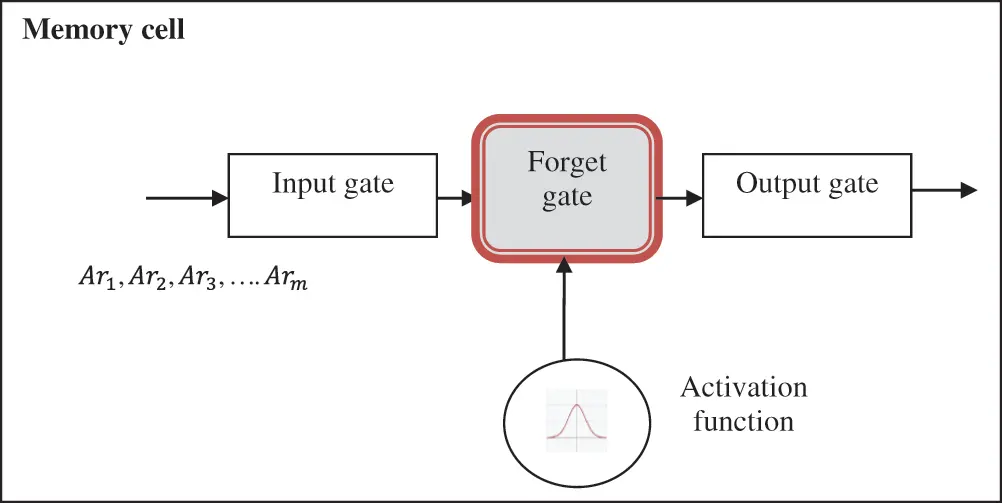Figure 3: Internal structure of RKLSTM

Fig. 4 depicts the structural design of the weighted sum of the input. The attribute selection process of the forget gate is expressed as follows:

F(t)=σR(ωi(t)βi+ρfh(t1)+z) (1)

where F(t) indicates the forget gate output at time instance t . For each input, Z denotes a bias that it is a constant, thereby helping the network to fit best for the given input data. Bias is an additional input to the input and it has a constant value of 1, ωi(t) denotes a current input and βi and ρf indicate a weight matrix, h(t1) denotes a previous layer output, and σR indicates an activation function. The radial basis kernel activation function is applied to find similar attributes as follows:

σR=e[AriArj22D2] (2)

where σR indicates an activation function, D denotes a deviation, Ari and Arj denote the attributes, and indicates a distance similarity between the attributes. The radial basis kernel activation function is responsible for making a decision to which values are stored or discarded in cell state. Radial basis is an activation function offers two outcomes: 0 and 1. An outcome of 0 denotes that RKLSTM forgets the particular results, and 1 means that the forget gate remembers information at a particular time step. That is, the forget gate displays significant attributes for network traffic prediction and discard other attributes. The attribute selection process of the proposed RKLSTM-CTMDSL model reduces the traffic prediction time and space complexity.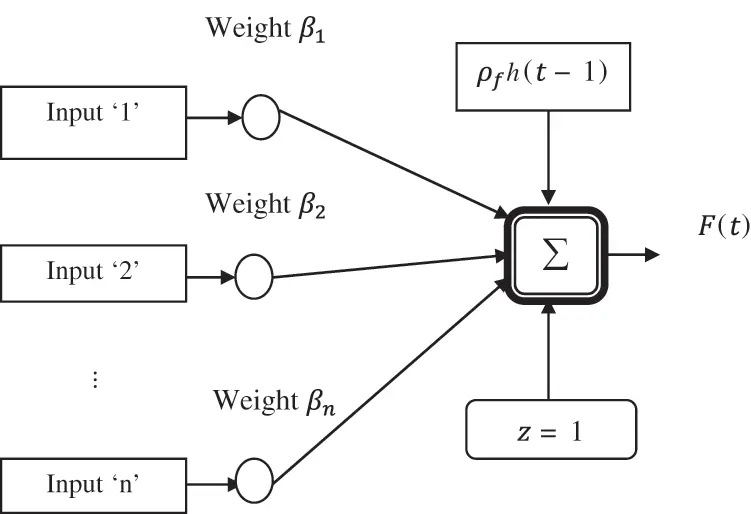Figure 4: Structural design of the weighted sum of the input

Thereafter, the selected attributes are forwarded to the next hidden layer for classifying data. Data for the selected attributes are taken for classification. The Tversky index calculates similarity among data and network traffic patterns. A similarity measure is the most significant tool in traffic pattern classification. A similarity measure is used to determine the correlation between two variables with a numerical value. Therefore, the Tversky similarity index is measured as follows:

ST=ditpτ(diΔtp)+ϑ(ditp), (3)

where ST denotes a Tversky similarity coefficient, di signifies the network data, tp indicates the network traffic patterns, ditp denotes mutual dependence between the two variables, and diΔtp denotes a variance between the variables. From (3), τ and ϑ indicate the parameters of the Tversky index ( τ,ϑ0 ). The similarity coefficient ( ST ) offers value among [0,1]. Similarity results are given to the output layer of deep structure learning.

Y(t)={ST0.5HeavynetworktrafficST0.5normalnetworktraffic, (4)

where Y(t) denotes an output and ST indicates a similarity value. Similarity value above 0.5 is classified as heavy network traffic; otherwise, traffic is classified as normal network traffic. The predicted result displays the output results and computes the error to determine the correct prediction and minimizes the incorrect prediction. The error rate is calculated as follows:

Et=[(Ya(t))(Y(t))]2, (5)

where Et denotes a training error, Ya(t) denotes an actual result, and Y(t) denotes predicted results. After identifying the training error, the weights are adjusted until it finds the minimum training error. The proposed RKLSTM-CTMDSL model uses gradient descent function to discover minimal prediction error.

f(x)=argminEt, (6)

where f(x) is a gradient descent function, argmin denotes argument of minimum function, and Et is a training error. Thus, network traffic prediction is performed by the RKLSTM-CTMDSL model. The algorithmic procedure of proposed RKLSTM-CTMDSL model is described as follows.

Algorithm 1 explains the process of cellular traffic prediction with minimum time consumption. The deep structure learning architecture receives the number of attributes and data in the input layer. Thereafter, inputs are transferred into a hidden layer. In the hidden layer, attribute selection is performed by applying RKLSTM, which has three gates. The input gate receives the attributes and analyzes the attributes in the forget gate with the help of the radial basis kernel activation function. The radial basis kernel activation function analyzes the attributes and displays the results at the output layer. Depending on the activation function outcome, additional related attributes are selected for classification. The remaining attributes are rejected from the data set. With the selected significant attributes, classification is performed using the Tversky similarity index. Similarity is computed among network data and traffic patterns. If data that are similar to the network patterns are classified as heavy network traffic. Otherwise, data are classified as normal network traffic. Lastly, traffic prediction outcomes are attained. Error is likewise measured for each predicted result at the output layer to minimize incorrect classification results. The gradient descent function finds superior prediction results.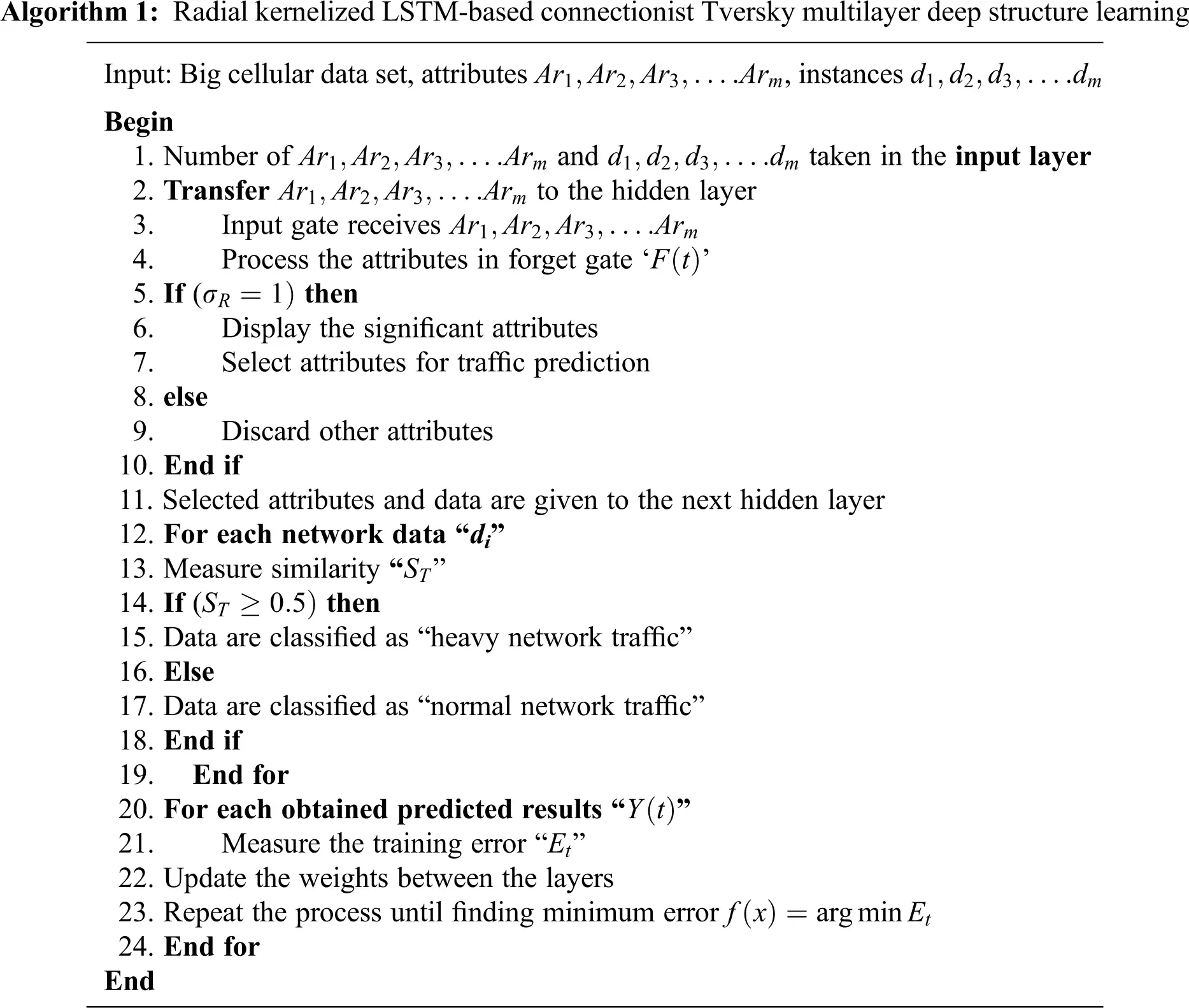4  Experimental Setup and Parameter Evaluation

The performance of the RKLSTM-CTMDSL model and conventional methods, namely, graph-based deep learning approach  and STCNet , are executed in Java. To conduct the experimental evaluation, city-cellular-traffic-map data set (https://github.com/caesar0301/city-cellular-traffic-map) is used. This data set includes timestamp and location information gathered from cellular base stations (BS). This data set comprises different trace files, namely, traffic and topology. Traffic trace file includes 1625680 instances and 5 columns (i.e., attributes). Topology file includes 13296 instances and 3 attributes. Thereafter, the large-volume spatiotemporal data are gathered for traffic prediction. The experimental process of RKLSTM-CTMDSL and existing methods are carried out with various parameters. The descriptions of different metrics are presented as follows.

•   Prediction Accuracy ( PA ): PA is calculated as the number of spatiotemporal data is classified into two different classes, namely, normal or heavy network traffic to the total number of spatiotemporal data. PA is calculated as follows:

PA=[zECk]100, (7)

where PA indicates the prediction accuracy, zEC is the number of data correctly classified, k denotes the total number of spatiotemporal data, and PA is calculated in percentage (%).

•   Error rate (ER): ER is measured as the number of spatiotemporal data is inaccurately predicted as normal or heavy network traffic. The error rate is mathematically computed as follows:

ER=[zICk]100, (8)

where ER specifies the error rate, zIC designates the number of inaccurately classified, and k denotes the total number of spatiotemporal data. ER is calculated in percentage (%).

•   Prediction time (PT): PT is measured as the amount of time consumed to predict normal or heavy traffic with cellular data. Therefore, PT is given as follows:

PT=[EDtime][STtime], (9)

where EDtime denotes an ending time of spatiotemporal data classification and STtime designates the starting time of spatiotemporal data classification. PT is calculated in milliseconds (ms).

•   Space complexity ( CompSp ): Space complexity is the significant metric used to discover the amount of memory space taken by the algorithm to store traffic data for prediction. The formula for space complexity is expressed as follows:

CompSp=kMem[SSd], (10)

where CompSp is the space complexity, k is the number of data, Mem denotes memory consumption, and SSd denotes storing the single data. Memory consumption is measured in megabytes (MB).

Tab. 1 illustrates the traffic prediction accuracy results of the RKLSTM-CTMDSL model, graph-based deep learning approach , and STCNet , with the number of spatiotemporal data ranging from 1000 to 10000. From the quantitative analysis, the RKLSTM-CTMDSL model significantly outperforms the other methods. The RKLSTM-CTMDSL model achieves 88% accuracy by applying 1000 spatiotemporal data. Similarly, the traffic prediction accuracies of [1,2] are 78% and 69%, respectively, by applying similar counts of input data. The discussion shows that the RKLSTM-CTMDSL model correctly classified 880 spatiotemporal data, and 780 and 690 data are accurately classified by the two methods [1,2]. The prediction accuracy of the RKLSTM-CTMDSL model is increased by 7% compared with Wang et al.  and 18% compared with Zhang et al. .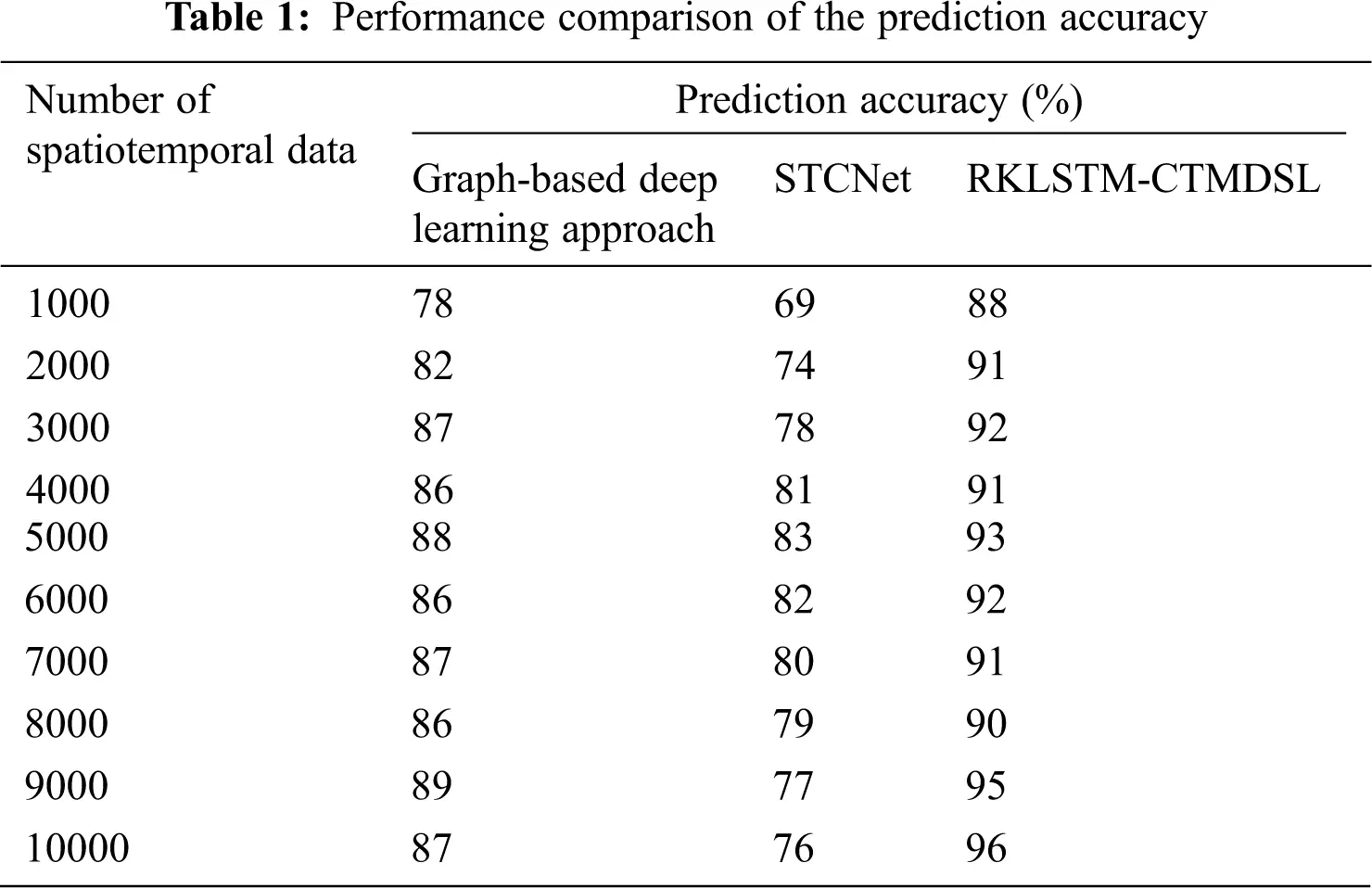Fig. 5 illustrates the prediction accuracy of the three methods. The curves indicate that the proposed model achieves higher accuracy than the other two methods. This significant improvement of the proposed technique is achieved by applying the connectionist Tversky indexing multilayer deep structure learning. Similarity function analyzes the attribute value with the testing traffic patterns. Thereafter, the normal or heavy network traffic data are correctly classified based on similarity value, and the results are shown in the output layer.Figure 5: Graphical illustration of the prediction accuracy

Tab. 2 shows the performance of the error rate of the three methods with the number of spatiotemporal data. The reported results in Tab. 2 indicate that the error rate is minimized using the RKLSTM-CTMDSL model compared with the conventional deep learning approaches. The proposed deep structure learning accurately matches the input data with the traffic patterns. Training error is measured after predicting the results. Thereafter, the gradient descent function discover error minimized network prediction results. The graphical plots of the three deep learning methods are illustrated in Fig. 6.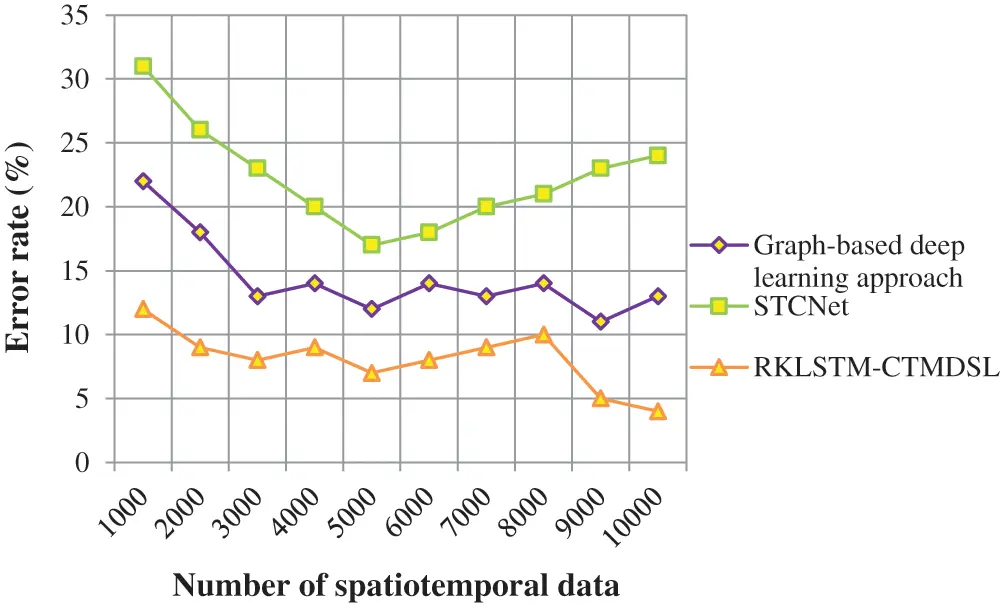Figure 6: Graphical illustration of the error rate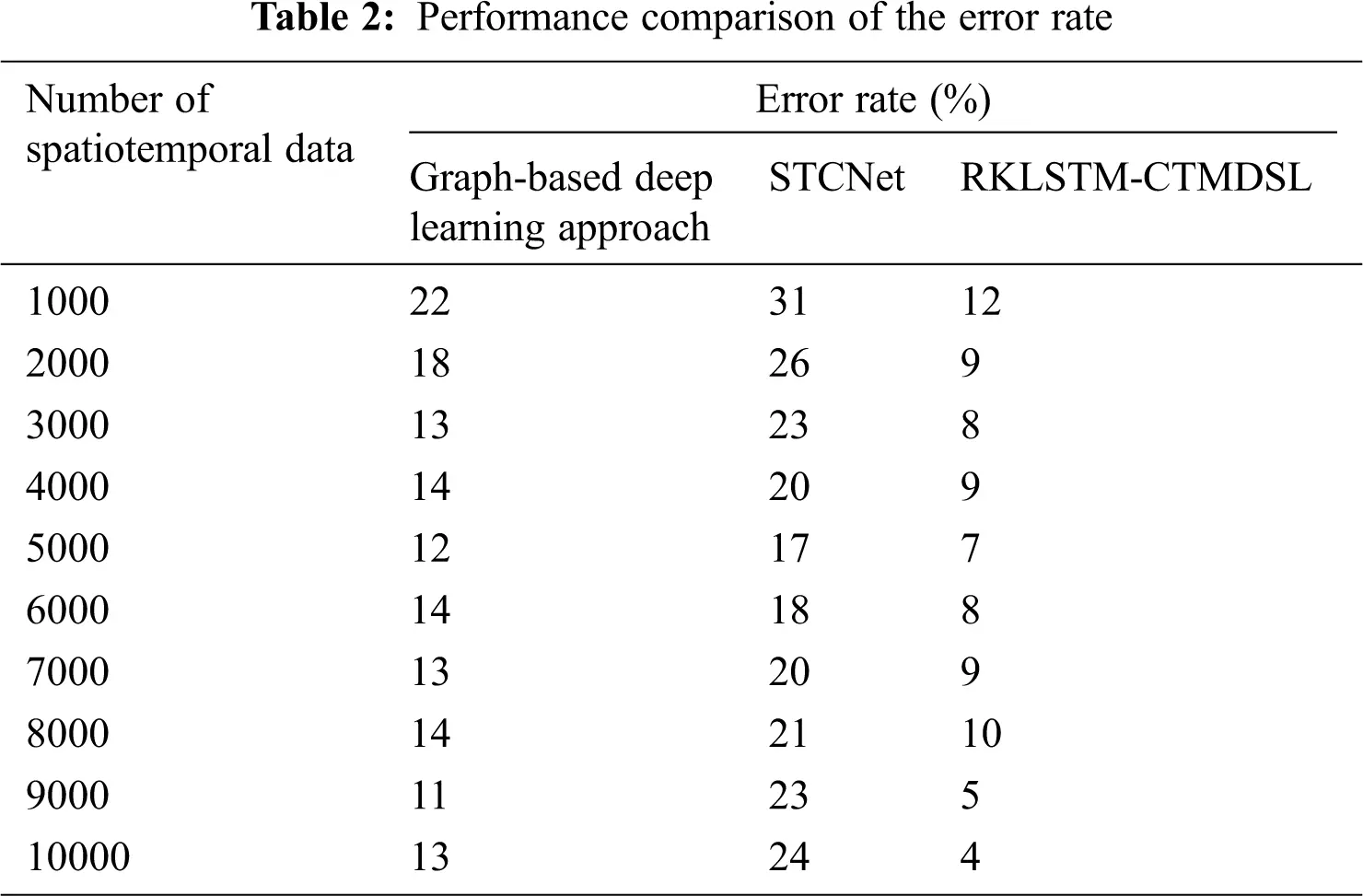Fig. 6 demonstrates an illustrative performance of the error rate in the cellular traffic prediction. This experiment illustrates the errors of all the methods affected by traffic volume prediction. As shown in Fig. 6, the graphical plots illustrate the error rate of the cellular network traffic prediction. The average of the 10 results indicates that the error rate is comparatively less by 44% using the RKLSTM-CTMDSL model compared with Wang et al. , and also minimized by 63% compared with Zhang et al. .

The comparative analysis of the prediction time versus the number of spatiotemporal big data is shown in Tab. 3. The reported results confirm that the RKLSTM-CTMDSL model achieves less prediction time than the other two approaches. This result is proven through the statistical evaluation by considering 1000 input spatiotemporal cellular network data. From the observation, the prediction time of the RKLSTM-CTMDSL model achieves 15ms, and the time taken by the other two approaches [1,2] are observed 17ms and 20ms , respectively. Similarly, the different prediction times are observed. The cellular network traffic prediction time of the RKLSTM-CTMDSL model is reduced by 10% and 16% compared with the existing deep learning approaches.Fig. 7 shows the results of the prediction time using three methods. As demonstrated in the preceding graphical plot, the prediction time curves are gradually increased when increasing the input counts of the spatiotemporal data. Among the three methods, the RKLSTM-CTMDSL model reduces the prediction time. This improvement is achieved by selecting the significant attributes and discarding the other attributes. The RKLSTM-CTMDSL model uses the radial kernelized LSTM for finding similar attributes from the data set. Given the limited number of significant attributes, the traffic prediction performed results in the RKLSTM-CTMDSL model reducing the time consumption compared with the other existing approaches.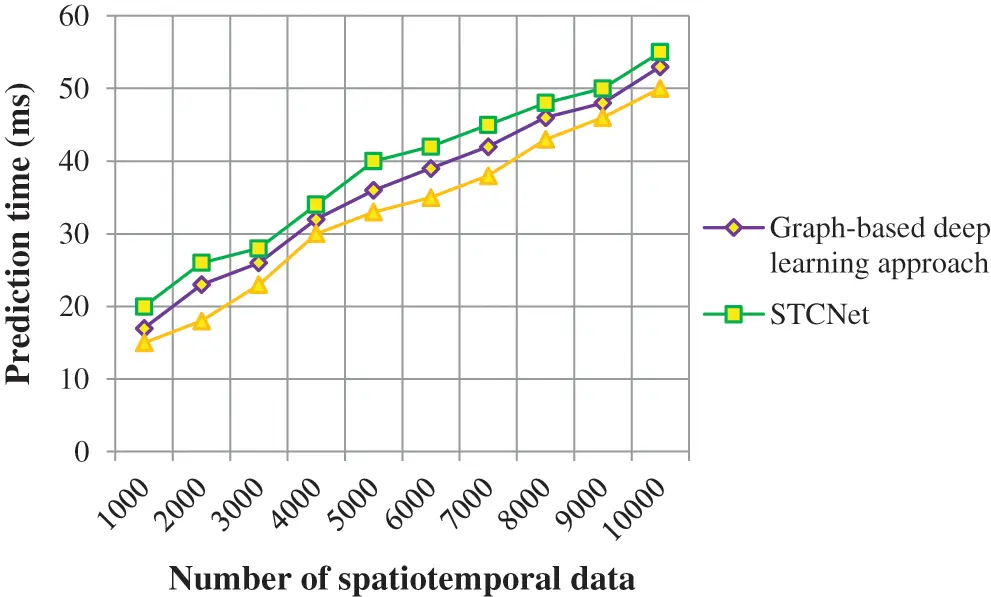Figure 7: Graphical illustration of the prediction time

Tab. 4. and Fig. 8 show the amount of memory space involved in storing big spatiotemporal data ranging from 1000 to 10000. That is, with an enhanced number of spatiotemporal data, space complexity also increases because the data set comprises the different lengths of the data. The memory consumption of the RKLSTM-CTMDSL model is minimized compared with the other two approaches. However, it is observed from the sample calculations. Consider the 1000 data for calculating the memory consumption. First, the RKLSTM-CTMDSL model consumes 18 MB of memory for storing the data. Second, the memory consumption of [1,2] is observed by 20 MB and 25 MB, respectively. The dimensionality reduction of the data set facilitates a decrease in space complexity. The dimensionality reduction of the data set is achieved by choosing the significant attributes for traffic prediction. Consequently, the space complexity incurred using the RKLSTM-CTMDSL model is found minimized by 10% compared with the graph-based deep learning approach  and by 21% compared with STCNet .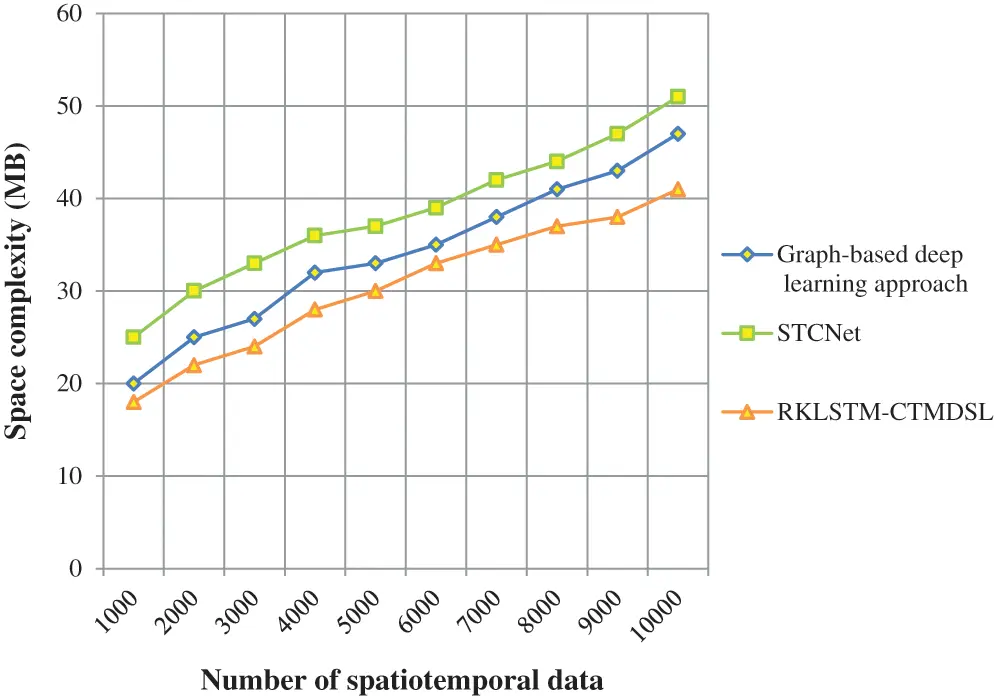Figure 8: Graphical illustration of the space complexity5  Conclusion

RKLSTM-CTMDSL is introduced to attain superior accuracy with time and memory consumptions. Extensive traffic information is initially collected from the data set and given to the hidden layer for learning the attributes. Radial basis kernelized LSTM is applied to learn the multiple attributes and identify the related attributes for reducing the time consumption with the help of activation function. Tversky index is applied in the hidden layer to analyze the training data with the traffic test data. The results are given to the output layer and display prediction results. A sequence of experimental evaluation is conducted using a city-cellular-traffic-map data set. Experimental validation is used to demonstrate the performance of the RKLSTM-CTMDSL model compared with the existing approaches. The quantitative results verified that the RKLSTM-CTMDSL model turns in superior performance in terms of traffic prediction accuracy compared with conventional methods.

Funding Statement: The authors received no specific funding for this study.

Conflicts of Interests: The authors declare they have no conflicts of interest to report regarding this study.

## References

1. X. Wang, Z. Zimu, X. Fu and X. Kai, “Spatio-temporal analysis and prediction of cellular traffic in metropolis,” IEEE Transactions on Mobile Computing, vol. 18, no. 9, pp. 2190–2202, 2018.
2. C. Zhang, Z. Haixia, Q. Jingping and Y. Dongfeng, “Deep transfer learning for intelligent cellular traffic prediction based on cross-domain big data,” IEEE Journal on Selected Areas in Communications, vol. 37, no. 6, pp. 1389–1401, 2019.
3. J. Mejia, O. Z. Alberto and C. M. Oliverio, “Traffic forecasting on mobile networks using 3D convolutional layers,” Mobile Networks and Applications, vol. 25, pp. 2134–2140, 2020.
4. K. Zhang, C. Gang, G. Weidong and L. Xuewen, “A new method for traffic forecasting in urban wireless communication network,” EURASIP Journal on Wireless Communications and Networking, vol. 219, no. 66, pp. 1–12, 2019.
5. R. Shinkuma, T. Yusuke, Y. Yoshinobu and T. Eiji, “User instruction mechanism for temporal traffic smoothing in mobile networks,” Computer Networks, vol. 137, pp. 17–26, 2018.
6. L. N. Wang, Z. C. Rui and C. Y. Yuan, “The short-term prediction of the mobile communication traffic based on the product seasonal model,” SN Applied Sciences, vol. 2, no. 3, pp. 1–9, 2020.
7. C. Qiu, Z. Yanyan, F. Zhiyong and Z. Ping, “Spatio-temporal wireless traffic prediction with recurrent neural network,” IEEE Wireless Communications Letters, vol. 7, no. 4, pp. 554–557, 2018.
8. D. Zhang, L. Linan, X. Cheng and Y. Bing, “Citywide cellular traffic prediction based on a hybrid spatiotemporal network,” Algorithms, vol. 13, no. 1, pp. 1–16, 2020.
9. L. Rongpeng, Z. Zhifeng, Z. Jianchao, M. Chengli, Y. Cai et al., “The learning and prediction of application-level traffic data in cellular networks,” IEEE Transactions on Wireless Communications, vol. 16, no. 6, pp. 3899–3912, 2017.
10. C. Zhang, Z. Haixia, Y. Dongfeng and Z. Minggao, “Citywide cellular traffic prediction based on densely connected convolutional neural networks,” IEEE Communications Letters, vol. 22, no. 8, pp. 1656–1659, 2018.
11. K. Fanhui, J. Li, B. Jiang and T. Zhang, “Big data-driven machine learning-enabled traffic flow prediction,” Transactions on Emerging Telecommunications Technologies, Wiley, vol. 30, no. 9, pp. 1–13, 2019.
12. Z. Liu, R. Wang and D. Tang, “Extending labeled mobile network traffic data by three levels traffic identification fusion,” Future Generation Computer Systems, vol. 88, pp. 453–466, 2018.
13. L. Chen, Y. Dingqi, Z. Daqing, W. Cheng, T. M. T. Nguyen et al., “Deep mobile traffic forecast and complementary base station clustering for C-RAN optimization,” Journal of Network and Computer Applications, vol. 121, pp. 59–69, 2018.
14. Z. Shuang, S. Chen, Y. Sun and Z. Cai, “Identifying known and unknown mobile application traffic using a multilevel classifier,” Security and Communication Networks, vol. 2019, pp. 1–12, 2019.
15. S. A. Abdulkarim and I. A. Lawal, “A cooperative neural network approach for enhancing data traffic prediction,” Turkish Journal of Electrical Engineering and Computer Sciences, vol. 25, no. 6, pp. 4746–4756, 2017.
16. F. Xu, Y. Li, H. Wang, P. Zhang, D. Jin et al., “Understanding mobile traffic patterns of large scale cellular towers in urban environment,” IEEE/ACM Transactions on Networking, vol. 25, no. 2, pp. 1147–1161, 20
17. F. Luoyang, X. Cheng, H. Wang and L. Yang, “Mobile demand forecasting via deep graph-sequence spatiotemporal modeling in cellular networks,” IEEE Internet of Things Journal, vol. 5, no. 4, pp. 3091–3101, 2018.
18. F. Xu, Y. Lin, J. Huang and D. Wu, “Big data driven mobile traffic understanding and forecasting: A time series approach,” IEEE Transactions on Services Computing, vol. 9, no. 5, pp. 796–805, 2016.
19. C. W. Huang and P. C. Chen, “Joint demand forecasting and DQN-based control for energy-aware mobile traffic offloading,” IEEE Access, vol. 8, pp. 66588–66597, 2020.
20. Y. Yamada, S. Ryoichi, T. Iwai and T. Onishi, “Temporal traffic smoothing for IoT traffic in mobile networks,” Computer Networks, vol. 146, pp. 115–124, 2018.This work is licensed under a Creative Commons Attribution 4.0 International License, which permits unrestricted use, distribution, and reproduction in any medium, provided the original work is properly cited.